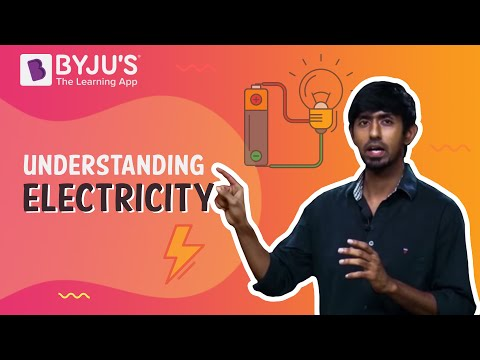Jet Set Go! All about Aeroplanes Jet Set Go! All about Aeroplanes

# Relation Between Watt And Volt

Named after James Watt, Watt is the unit of power. In terms of electromagnetism, it is the current flow of one ampere with a voltage of 1 volt. A volt is a derived unit for electric potential, electromotive force, and electric potential difference. The relation between watt and volt is direct. This implies that a change in the value of watt will reflect as a change in the value of volt.

## Watt And Volt

In physics, the relation between watt and volt can be written as:

1 watt = 1 ampere × 1 volt

$$\begin{array}{l}1\;volt=\frac{1\;watt}{1\;ampere}\end{array}$$

Where,

• Watt is the measure of power.
• Volt is the measure of electric potential.
• Ampere is the measure of current.

 Volt is the measure of potential difference within two terminal of a conducting wire. Watt is the rate at which electrical work is performed when a current of one ampere flows across the potential difference of one volt.

The relation between watt and volt is proportionate. That is watt is directly proportional to volt. Which implies that-

• When the electric power in terms of watt increases, the electric potential in terms of volt also increases, keeping the electric current constant.
• When the electric power in terms of watt decreases, the electric potential in terms of volt also decreases keeping the electric current constant.

### AC and DC

The formula for watt and volt changes for alternating current and direct current is given below:

 Current Type Formula Terms AC PW= PF × VV × IA PW is Power in watt PF is Power Factor VV is voltage in volts IA is current in ampere DC PW= VV × IA PW is Power in watt VV is voltage in volts IA is current in ampere

Hope you have understood the relation between watt and volt, the units of electricity. Also, watt and volt conversion in physics, electronics especially in electromagnetism.

Similar Articles:

Stay tuned with BYJU’S for more such interesting articles. Also, register to “BYJU’S-The Learning App” for loads of interactive, engaging physics-related videos and an unlimited academic assist.Test Your Knowledge On Relation Between Watt And Volt!